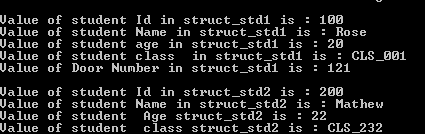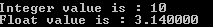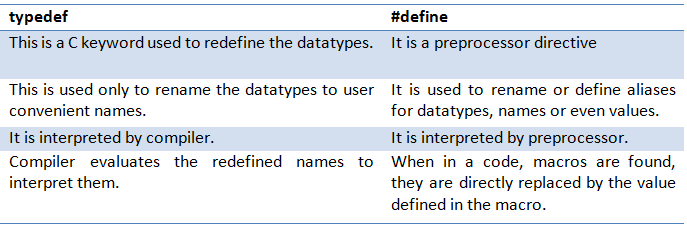Typedef in C Programming

We have seen how to declare structures and unions, and to initialize and access them. Sometimes in the program we might have to use the same structure / union in multiple places – across different functions. In such cases we might end up in creating the same structure for these structures/unions again and again. Instead we can create user-defined datatype for these structures/ unions at the beginning and can use this newly created datatype anywhere in the program / function to declare the variables.

For example consider a program, which contains one function to accept student details from the user and another function to display the values in structure. Now both these function access the same structure skeleton to accept the data as well as to print the data. Hence we have create the skeleton of the student structure first and then we have to create structure variable in all the function to access them.

struct student {
int intStdId;
char chrStdName ;
int intAge;
char chrClassId ;
};

When we create structure variable for student, we end up in writing as below multiple times across the functions

struct student struct_std;

Instead of typing above big lines multiple times in all the functions, we can create user defined datatype for structure student by using typedef.

i.e.;

typedef struct student {

int intStdId;
char chrStdName ;
int intAge;
char chrClassId ;
} struct_student;

Now struct_student can be used all the function as a datatype to declare a structure variable of the type student.

i.e.;     struct_student struct_std;

#include
#include

typedef struct student{
int intStdId;
char chrStdName;
int intAge;
char chrClassId;
int intDoorNum;
char chrstreet;
char chrCity;
char chrState;
int intPincode;
};
}struct_std;
void main() {
struct_std struct_std1, struct_std2; //Instead of  'struct student struct_std1, struct_std2' user defined name is used as datatype

struct_std1.intStdId = 100; 	strcpy(struct_std1.chrStdName, "Rose");
struct_std1.intAge = 20;	strcpy(struct_std1.chrClassId, "CLS_001");
struct_std1.intDoorNum = 121;	strcpy(struct_std1.chrState, "Bangalore");

struct_std2.intStdId = 200; 	strcpy(struct_std2.chrStdName, "Mathew");
struct_std2.intAge = 22;	strcpy(struct_std2.chrClassId, "CLS_232");

printf("\nValue of student Id in struct_std1 is : %d", struct_std1.intStdId);
printf("\nValue of student Name in struct_std1 is : %s", struct_std1.chrStdName);
printf("\nValue of student age in struct_std1 is : %d", struct_std1.intAge);
printf("\nValue of student class  in struct_std1 is : %s", struct_std1.chrClassId);
printf("\nValue of Door Number in struct_std1 is : %d", struct_std1.intDoorNum);

printf("\nValue of student Id in struct_std2 is : %d", struct_std2.intStdId);
printf("\nValue of student Id in struct_std2 is : %s", struct_std2.chrStdName);
printf("\nValue of student Id in struct_std2 is : %d", struct_std2.intAge);
printf("\nValue of student Id in struct_std2 is : %s", struct_std2.chrClassId);
}We can use the same method to define unions too. For unions also, it will create a user defined datatypes to declare them again and again in the program and across the functions

Identifiers in C Programming

C allows simple to complex datatypes in its program. Complex datatypes include arrays, pointers, structures, unions etc. In the case of complex datatypes, if we need to use them repeatedly in the program, then it is tedious to write them again and again. Instead, if we have user defined datatype for these complex ones, then it is easy to type, understand and use. This is done by using the command ‘typedef’.

It allows users to redefine the name for the datatype. For example, student structure where student Id, student name, age and address are there.

struct Student {
int StdId;
char StdName;
int age;
}STD;

Imagine we need to use same structure in different functions in the program. In such we have to define the structure of student structure every time.

struct Student std1, std2; // declaring a variable of type Student

Instead if we declare it with typedef, then we can declare student structure everywhere without defining all its structure everywhere.

typedef struct Student {
int StdId;
char StdName;
int age;
}STD

STD std1, std2; // declaring a variable of type Student

Now it is easy to declare structure variables. In above case, we need not write full ‘struct Student std1, std2; ‘ to declare std1 and std2 of type Student. Instead ‘STD’ itself denotes whole ‘struct Student’. There is no difference in accessing structure elements. Here only the difference is in declaring the structure type variable. This typedef can be used to declare variable of any type. Suppose we have variables declared using simple datatypes. If the user wants to rename it to some other convenient name, then he can do it using typedef.

Storage Classes in C Programming

typedef unsigned int INT; // now INT can be used to declare a variable of type unsigned int in the current program
INT intNum;

typedef float FLOAT;
FLOAT pi;

For convention and for better understanding, uppercase is used to define the userdefined datatypes. We can even lower case for the same. But upper case makes user easily understand that it is a typedef datatype.

typedef float floats;
floats pi;

#include
typedef unsigned int INTEGER; // now INTEGER can be used to declare a variable of type unsigned int in the current program
typedef float FLOAT;

void main (){
INTEGER intNum = 10;//user defined datatype INTEGER
FLOAT pi = 3.14; //user defined datatype FLOAT

printf("Integer value is : %d\n", intNum);
printf("Float value is : %f", pi);
}The preprocessor directive ‘#define’ also provides the similar functionality. It also can be used to redefine the datatype to user convenient way. But it has few differences from typedef.